Function Repository Resource:

# Cofactor

Get a cofactor of a matrix

Contributed by: Wolfram Staff
 ResourceFunction["Cofactor"][m,{i,j}] gives the (i,j) cofactor of the square matrix m.

## Details

The (i,j) cofactor of a square matrix is, up to a change in sign, equal to the determinant of the matrix obtained by deleting the ith row and jth column.

## Examples

### Basic Examples (2)

Here is the (1, 2) cofactor of a 3×3 matrix:

 In:=Out=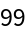The positively signed cofactor of the top left-hand corner of an array:

 In:=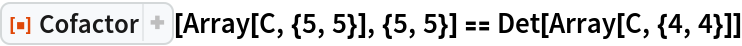Out=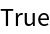### Scope (4)

Define a random 4×4 matrix:

 In:=Out=Here is its determinant:

 In:=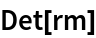Out=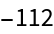Expand the determinant along the first row:

 In:=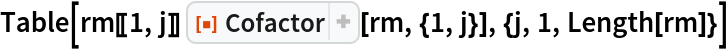Out=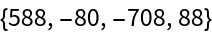Adding up gives the determinant again:

 In:=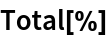Out=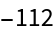### Properties and Relations (7)

A 3×3 matrix:

 In:=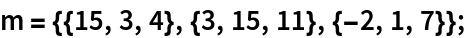Compute its cofactor matrix using the resource function CofactorMatrix:

 In:=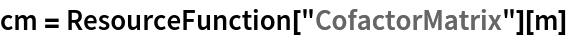Out=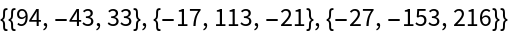The (i,j) cofactor is equal to the (i,j)th element of the cofactor matrix:

 In:=Out=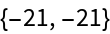In:=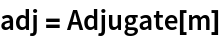Out=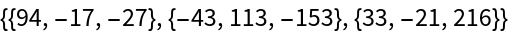The (i,j) cofactor is equal to the (j,i)th element of the adjugate:

 In:=Out=Compute its matrix of minors:

 In:=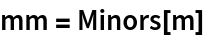Out=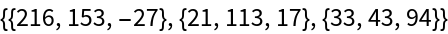The (i,j) cofactor is, up to a change in sign, equal to the (n-i+1,n-j+1)th element of the matrix of minors:

 In:=Out=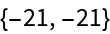George Beck

## Version History

• 1.0.1 – 31 January 2022
• 1.0.0 – 05 July 2019